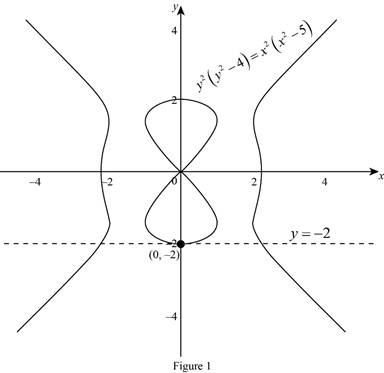# The equation of the tangent line to the given equation at the point.### Single Variable Calculus: Concepts...

4th Edition
James Stewart
Publisher: Cengage Learning
ISBN: 9781337687805### Single Variable Calculus: Concepts...

4th Edition
James Stewart
Publisher: Cengage Learning
ISBN: 9781337687805

#### Solutions

Chapter 3.5, Problem 28E
To determine

## To find: The equation of the tangent line to the given equation at the point.

Expert Solution

The equation of the tangent line to the equation y2(y24)=x2(x25) at the point (0,2) is y=2.

### Explanation of Solution

Given:

The curve is y2(y24)=x2(x25).

The point is (0,2).

Derivative rules:

(1) Chain rule: If y=f(u) and u=g(x)  are both differentiable function, then dydx=dydududx.

(2) Product rule: ddx(f(x)g(x))=f(x)ddx(g(x))+g(x)ddx(f(x))

Formula used:

The equation of the tangent line at (x1,y1) is, yy1=m(xx1) (1)

Where, m is the slope of the tangent line at (x1,y1) and m=dydx|x=x1,y=y1.

Calculation:

Obtain the equation of the tangent line to the equation at the point.

Rewrite the given equation as follows,

y2(y24)=x2(x25)y44y2=x45x2

Differentiate the equation implicitly with respect to x,

ddx(y44y2)=ddx(x45x2)ddx(y4)4ddx(y2)=ddx(x4)5ddx(x2)ddx(y4)4ddx(y2)=4x210x

Apply the chain rule (1) and simplify the terms,

[ddy(y4)dydx]4[ddy(y2)dydx]=4x210x4y3dydx4[2ydydx]=4x210x4y3dydx8ydydx=4x210x

Substitute (0,2) for (x,y),

4(2)3dydx8(2)dydx=4(0)210(0)(4)8dydx+16dydx=0dydx=0

Thus, the slope of the tangent at (0,2) is m=0.

Substitute (0,2) for (x1,y1) and m=0 in equation (1),

y+2=0(x0)y+2=0y=2

Therefore, the equation of the tangent line to the equation y2(y24)=x2(x25) at (0,2) is y=2.

Graph:

The graph of the given curve and tangent line is shown below Figure 1.From Figure 1, it is observed that the line y=2 is tangent to the equation y2(y24)=x2(x25) at the point (0,2).

### Have a homework question?

Subscribe to bartleby learn! Ask subject matter experts 30 homework questions each month. Plus, you’ll have access to millions of step-by-step textbook answers!